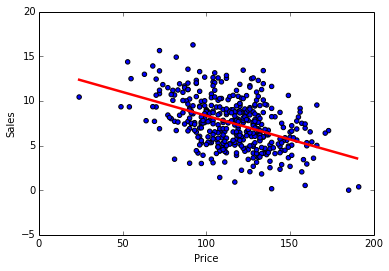Chapter 3: Linear Regression¶

Simple Linear Regression¶

In :
import pandas as pd
import numpy as np
import scipy as sp
import statsmodels.api as sm
import matplotlib.pyplot as plt
from sklearn.linear_model import LinearRegression
%matplotlib inline
In :
# Data from R ISLR package - write.csv(Boston, "Boston.csv", col.names = FALSE)
Out:
crim zn indus chas nox rm age dis rad tax ptratio black lstat medv
0 0.00632 18 2.31 0 0.538 6.575 65.2 4.0900 1 296 15.3 396.90 4.98 24.0
1 0.02731 0 7.07 0 0.469 6.421 78.9 4.9671 2 242 17.8 396.90 9.14 21.6
2 0.02729 0 7.07 0 0.469 7.185 61.1 4.9671 2 242 17.8 392.83 4.03 34.7
3 0.03237 0 2.18 0 0.458 6.998 45.8 6.0622 3 222 18.7 394.63 2.94 33.4
4 0.06905 0 2.18 0 0.458 7.147 54.2 6.0622 3 222 18.7 396.90 5.33 36.2

5 rows × 14 columns

In :
# LSTAT - % of population with low status; MEDV - median value of home
ax = boston_df.plot(x="lstat", y="medv", style="o")
ax.set_ylabel("medv")
Out:
<matplotlib.text.Text at 0x4cbaf50>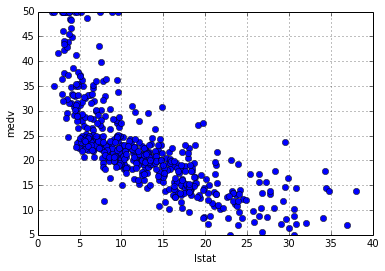In :
# The statsmodels library provides a small subset of models, but has more emphasis on
# parameter estimation and statistical testing. The summary output is similar to R's
# summary function.
# X is an "array" of column values, y is a single column value
X = boston_df[["lstat"]].values
X = sm.add_constant(X)  # add the intercept term
y = boston_df["medv"].values
ols = sm.OLS(y, X).fit()
ols.summary()
Out:
Dep. Variable: R-squared: y 0.544 OLS 0.543 Least Squares 601.6 Fri, 23 May 2014 5.08e-88 20:55:56 -1641.5 506 3287. 504 3295. 1
coef std err t P>|t| [95.0% Conf. Int.] 34.5538 0.563 61.415 0.000 33.448 35.659 -0.9500 0.039 -24.528 0.000 -1.026 -0.874
 Omnibus: Durbin-Watson: 137.043 0.892 0 291.373 1.453 5.36e-64 5.319 29.7
In :
# Scikit Learn provides a larger number of models, but has more of a Machine Learning POV
# and doesn't come with the statistical testing data shown above. However, it produces an
# identical linear model as shown below:
reg = LinearRegression()
X = boston_df[["lstat"]].values
y = boston_df["medv"].values
reg.fit(X, y)
(reg.intercept_, reg.coef_)
Out:
(34.553840879383131, array([-0.95004935]))
In :
# Drawing the regression line on top of the scatterplot
ax = boston_df.plot(x="lstat", y="medv", style="o")
ax.set_ylabel("medv")

lstats = boston_df["lstat"].values
xs = range(int(np.min(X[:,0])), int(np.max(X[:,0])))
ys = [reg.predict([x]) for x in xs]
ax.plot(xs, ys, 'r', linewidth=2.5)
Out:
[<matplotlib.lines.Line2D at 0x4cbae90>]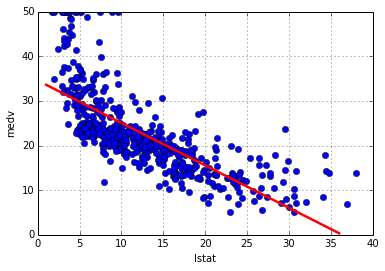In :
# Prediction
test_data = [, , ]
reg.predict(test_data)
Out:
array([ 29.80359411,  25.05334734,  20.30310057])

Multiple Linear Regression¶

In :
# regression with 2 input columns
X = boston_df[["lstat", "age"]]
reg2 = LinearRegression()
reg2.fit(X, y)
(reg2.intercept_, reg2.coef_)
Out:
(33.222760531792929, array([-1.03206856,  0.03454434]))
In :
# regression using all input columns
xcols = boston_df.columns[0:-1]
X = boston_df[xcols]
reg3 = LinearRegression()
reg3.fit(X, y)
(reg3.intercept_, reg3.coef_)
Out:
(36.459488385089394,
array([ -1.08011358e-01,   4.64204584e-02,   2.05586264e-02,
2.68673382e+00,  -1.77666112e+01,   3.80986521e+00,
6.92224640e-04,  -1.47556685e+00,   3.06049479e-01,
-1.23345939e-02,  -9.52747232e-01,   9.31168327e-03,
-5.24758378e-01]))
In :
# Plotting a fitted regression with R returns 4 graphs - Residuals vs Fitted, Normal Q-Q,
# Scale-Location (Standardized Residuals vs Fitted), and Residuals vs Leverage. Only the
# Q-Q plot is available from statsmodels. The residuals vs Fitted function is implemented
# below and is used for plot #1 and #3. The Residuals vs Leverage is TBD.
def residuals_vs_fitted(fitted, residuals, xlabel, ylabel):
plt.subplot(111)
plt.xlabel(xlabel)
plt.ylabel(ylabel)
plt.scatter(fitted, residuals)
polyline = np.poly1d(np.polyfit(fitted, residuals, 2))    # model non-linearity with quadratic
xs = range(int(np.min(fitted)), int(np.max(fitted)))
plt.plot(xs, polyline(xs), color='r', linewidth=2.5)

def qq_plot(residuals):
sm.qqplot(residuals)

def standardize(xs):
xmean = np.mean(xs)
xstd = np.std(xs)
return (xs - xmean) / xstd

fitted = reg3.predict(X)
residuals = y - fitted
std_residuals = standardize(residuals)

residuals_vs_fitted(fitted, residuals, "Fitted", "Residuals")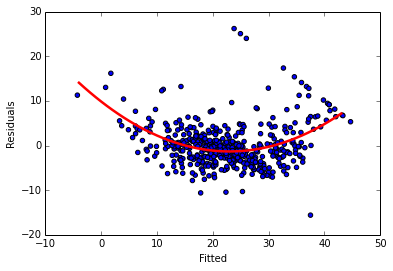In :
fig = sm.qqplot(residuals, dist="norm", line="r")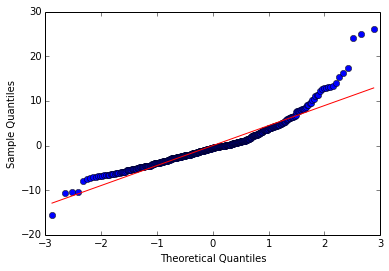In :
residuals_vs_fitted(fitted, std_residuals, "Fitted", "Std.Residuals")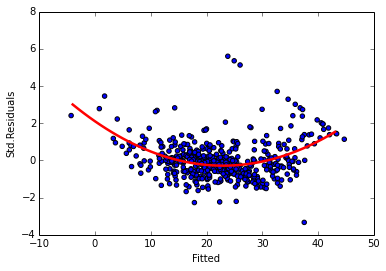Nonlinear Terms and Interactions¶

Python offers formula parsing support via the Patsy toolkit. StatsModels uses Patsy to provide formula parsing support for its models. But this can be easily implemented as temporary columns in Pandas dataframes as shown below.

In :
# fitting medv ~ lstat * age
boston_df["lstat*age"] = boston_df["lstat"] * boston_df["age"]
reg5 = LinearRegression()
X = boston_df[["lstat", "age", "lstat*age"]]
y = boston_df["medv"]
reg5.fit(X, y)
(reg5.intercept_, reg5.coef_)
Out:
(36.088535934612942,
array([ -1.39211684e+00,  -7.20859509e-04,   4.15595185e-03]))
In :
fitted = reg5.predict(X)
residuals = y - fitted
std_residuals = standardize(residuals)
residuals_vs_fitted(fitted, residuals, "Fitted", "Residuals")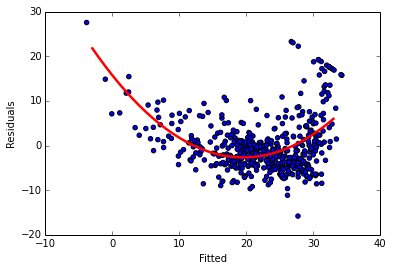In :
# fitting medv ~ lstat + I(lstat^2)
boston_df["lstat^2"] = boston_df["lstat"] ** 2
reg6 = LinearRegression()
X = boston_df[["lstat", "lstat^2"]]
y = boston_df["medv"]
reg6.fit(X, y)
# save the predicted ys for given xs for future plot
lstats = boston_df["lstat"].values
xs = range(int(np.min(lstats)), int(np.max(lstats)))
ys6 = [reg6.predict([x, x*x]) for x in xs]
(reg6.intercept_, reg6.coef_)
Out:
(42.862007328169383, array([-2.3328211 ,  0.04354689]))
In :
fitted = reg6.predict(X)
residuals = y - fitted
std_residuals = standardize(residuals)
residuals_vs_fitted(fitted, residuals, "Fitted", "Residuals")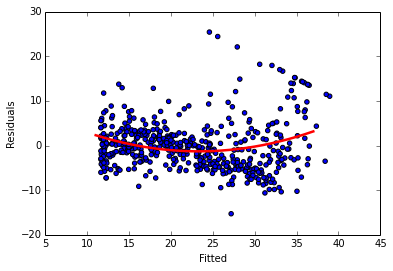In :
# fitting medv ~ poly(lstat,4). We already have lstat^2 and lstat from previous
boston_df["lstat^4"] = np.power(boston_df["lstat"], 4)
boston_df["lstat^3"] = np.power(boston_df["lstat"], 4)
X = boston_df[["lstat^4", "lstat^3", "lstat^2", "lstat"]]
y = boston_df["medv"]
reg7 = LinearRegression()
reg7.fit(X, y)
ys7 = [reg7.predict([x**4, x**3, x**2, x]) for x in xs]
(reg7.intercept_, reg7.coef_)
Out:
(46.800943987797865,
array([ -1.17511270e-05,  -1.17511460e-05,   9.23027375e-02,
-3.27115207e+00]))
In :
fitted = reg7.predict(X)
residuals = y - fitted
std_residuals = standardize(residuals)
residuals_vs_fitted(fitted, residuals, "Fitted", "Residuals")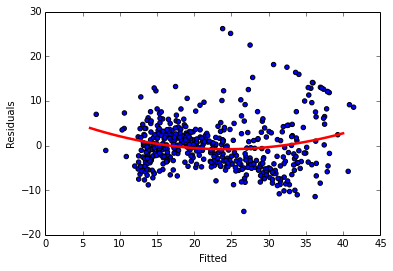In :
# Plot the different lines. Not that the green line (reg7) follows the distribution
# better than the red line (reg6).
ax = boston_df.plot(x="lstat", y="medv", style="o")
ax.set_ylabel("medv")
plt.plot(xs, ys6, color='r', linewidth=2.5)
plt.plot(xs, ys7, color='g', linewidth=2.5)
Out:
[<matplotlib.lines.Line2D at 0x56d1b50>]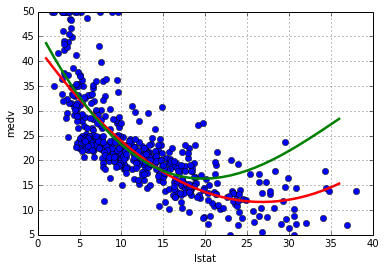Qualitative Predictors¶

In :
# Data from ISLR package: write.csv(Carseats, 'Carseats.csv', col.names=FALSE)
Out:
Sales CompPrice Income Advertising Population Price ShelveLoc Age Education Urban US
0 9.50 138 73 11 276 120 Bad 42 17 Yes Yes
1 11.22 111 48 16 260 83 Good 65 10 Yes Yes
2 10.06 113 35 10 269 80 Medium 59 12 Yes Yes
3 7.40 117 100 4 466 97 Medium 55 14 Yes Yes
4 4.15 141 64 3 340 128 Bad 38 13 Yes No

5 rows × 11 columns

In :
# convert non-numeric to factors
carseats_df["ShelveLoc"] = pd.factorize(carseats_df["ShelveLoc"])
carseats_df["Urban"] = pd.factorize(carseats_df["Urban"])
carseats_df["US"] = pd.factorize(carseats_df["US"])
# Sales ~ . + Income:Advertising + Age:Price
carseats_df["Age:Price"] = carseats_df["Age"] * carseats_df["Price"]
X = carseats_df[carseats_df[1:].columns]
y = carseats_df["Sales"]
reg = LinearRegression()
reg.fit(X, y)
(reg.intercept_, reg.coef_)
Out:
(5.773159728050814e-14,
array([  1.00000000e+00,  -7.59808882e-16,   1.38777878e-17,
5.55111512e-17,   3.25260652e-18,   4.02455846e-16,
2.22044605e-16,  -3.05311332e-16,  -4.16333634e-17,
-4.33680869e-18,  -3.64291930e-17,  -8.67361738e-18,
-1.22277147e-19]))
In :
# R has a contrasts() function that shows how factors are encoded by default. We can do
# this manually using scikit-learn's OneHotEncoder
from sklearn.preprocessing import OneHotEncoder

colnames = ["ShelveLoc", "Urban", "US"]
enc = OneHotEncoder()
X = carseats_df[colnames]
enc.fit(X)
X_tr = enc.transform(X).toarray()
colnos = enc.n_values_
colnames_tr = []
for (idx, colname) in enumerate(colnames):
for i in range(0, colnos[idx]):
colnames_tr.append(colname + "_" + str(i))
col = 0
for colname_tr in colnames_tr:
carseats_df[colname_tr] = X_tr[:, col]
col = col + 1
del carseats_df["ShelveLoc"]
del carseats_df["Urban"]
del carseats_df["US"]
Out:
ShelveLoc_0 ShelveLoc_1 ShelveLoc_2 Urban_0 Urban_1 US_0 US_1
0 1 0 0 1 0 1 0
1 0 1 0 1 0 1 0
2 0 0 1 1 0 1 0
3 0 0 1 1 0 1 0
4 1 0 0 1 0 0 1

5 rows × 7 columns

Writing Functions¶

We write a convenience function to plot a scatter plot and a regression line of two variables.

In :
def regplot(x, y, xlabel, ylabel, dot_style, line_color):
x = x.values
y = y.values
reg = LinearRegression()
X = np.matrix(x).T
reg.fit(X, y)
ax = plt.scatter(x, y, marker=dot_style)
plt.xlabel(xlabel)
plt.ylabel(ylabel)
xs = range(int(np.min(x)), int(np.max(x)))
ys = [reg.predict(x) for x in xs]
plt.plot(xs, ys, color=line_color, linewidth=2.5)

regplot(carseats_df["Price"], carseats_df["Sales"], "Price", "Sales", 'o', 'r')